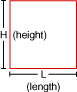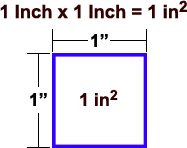Directions: Enter inches into the calculator below. You can use this square inches calculator to calculate siding square inches or any other square inches needed (like: roofing, carpet, etc.) You can also use this tool to convert inches to feet and calculate square feet.rectangle examples
and diagram

# Square Inches Calculator

(Height in Inches x Length in Inches = Square Inches)
(Square Inches = Sq In = Sq.In. = inches2 = in2)

inches    X

inches

## Directions:

Use the calculator above to calculate your square inches.
*Square Inches is also known as (a.k.a) square inch, square in, SqIn, Sq.In., sq.in., in2 & in2
**This Calculator also calculates square feet, total height in feet, total height in inches, total length in feet and total length in inches.## What is a Square Inch?

A "square inch" is a unit of area measurement equal to a square measuring one inch on each side. 1 Square Inch = 0.00064516 square meters. Abbrev.: in2, sq.in., SqIn.